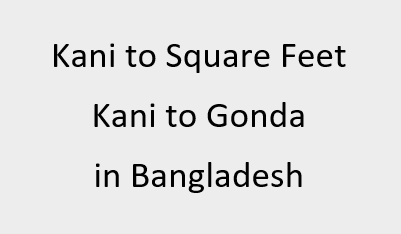# Kani to Square Feet | Kani to Gonda in Bangladesh## Kani to Square Feet | Kani to Gonda in BangladeshKani is a traditional unit of land measurement. It is used in the areas of southern Asia. The value of kani varies from region to region in which it is used. Here we will discuss the value of Kani as it is used in Bangladesh. It is also spelled as Koni, Kanni etc. Since it is not a standard unit people want to know the value of 1 kani is equal to how many square feet or kani is equal to how many acres or they want to convert kani to gonda or convert kani to sq ft.
20 Gonda make a kani.
1 Kani = 17424 Sq ft = 0.4 Acres = 1936 Sq. yards

Convert Kani to other units of Land area measurement :
 Value Convert from Til Kranti Kora Gonda Kani Sq. Centimeters Sq. Feet Sq. Yards Hectares Acres Sq. Meters Sq. Kilometers Sq. Miles Cent Convert to Til Kranti Kora Gonda Kani Sq. Centimeters Sq. Feet Sq. Yards Hectares Acres Sq. Meters Sq. Kilometers Sq. Miles Cent

Area Conversion of Kani to other Units and vice versa:
1 Kani = 4800 Til
1 Kani = 240 Kranti
1 Kani = 80 Kora
1 Kani = 20 Gonda
1 Kani = 0.4 Acre
1 Kani = 0.161874256896 Hectare
1 Kani = 17424 Sq. Feet
1 Kani = 1618.74256896 Sq. Meter
1 Kani = 0.00161874256896 Sq. Kilometer
1 Kani = 1936 Sq. Yard
1 Kani = 40 Decimal
1 Til = 0.000208333333333333 Kani
1 Kranti = 0.00416666666666667 Kani
1 Kora = 0.0125 Kani
1 Gonda = 0.05 Kani
1 Acre = 2.5 Kani
1 Hectare = 6.17763453667913 Kani
1 Sq. Feet = 5.73921028466483E-05 Kani
1 Sq. Meter = 0.000617763453667913 Kani
1 Sq. Kilometer = 617.763453667913 Kani
1 Sq. Yard = 0.000516528925619835 Kani
1 Decimal = 0.025 Kani

Q. 1 Kani = ? Chatak / Satak / Shotok
A. 1 Kani = 40 Chatak / Satak / Shotok

Q. 1 Kani is equal to how many square yards ?
A. 1 Kani = 1936 Square Yards

Q. What is the conversion between Kani and other traditional units ?
A. 1 Kani = 20 Gonda = 80 Kora = 240 Kranti = 4800 Til

Q. 1 Acre is equal to how many Kani ?
A. 1 Acre = 2.5 Kani